Using Green's theorem to Reduce a Surface Integration to a Path Integral

Suppose there is a uniformly charged surface  (σ≡charge/area=1) occupying an equilaterial triangle in the z=0 plane: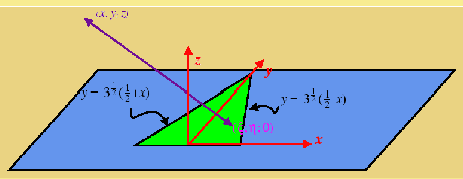The electrical potential goes like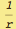, therefore the potential of a unit charge located at (x,y,z) from a small surface patch at (ξ,η,0) is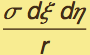=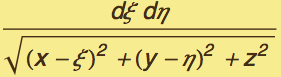Try to convert the integral over the region to an integral over its boundary.  We may be able to find a closed form—and even if not the numerical integration should be much more efficient...

We will use Green's Theorem in the Plane:
∫∫(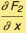-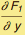)dxdy = ∫(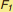dx +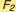dy)

Find a function, that when we take the derivative with respect to x, we get 1/r.
We will let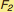be the function we find, and set=0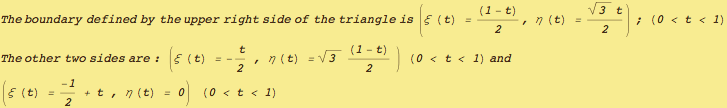To use Green's theorem in the plane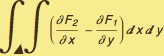=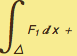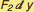Try to find a vector function (,) that matches the integrand over the domain.  Let= 0, then to find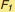which when differentiated by η gives 1/r

In:=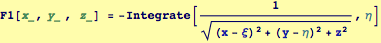Out=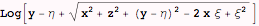The third (horizontal) boundary of the triangle patch looks like the easiest, let's see if an integral can be found over that patch:

In:=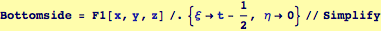Out=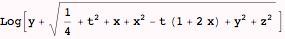The following attempt to find a closed-form integral seems to take "forever" to evaluate and I ended up aborting it, then continuing to evaluate the remainder of the notebook. This command has been commented out to avoid evaluation

In:=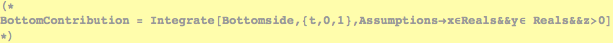In:=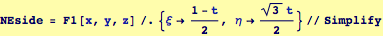Out=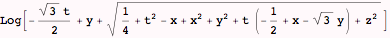In:=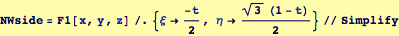Out=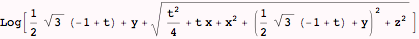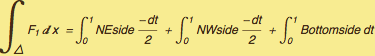In:=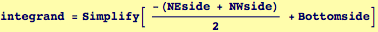Out=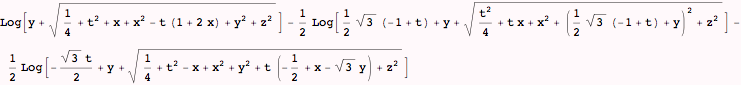The following attempt to find a closed-form integral seems to take "forever" to evaluate and I ended up aborting it, then continuing to evaluate the remainder of the notebook. This command has been commented out to avoid evaluation

In:=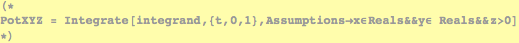Created by Wolfram Mathematica 6.0  (28 September 2007)